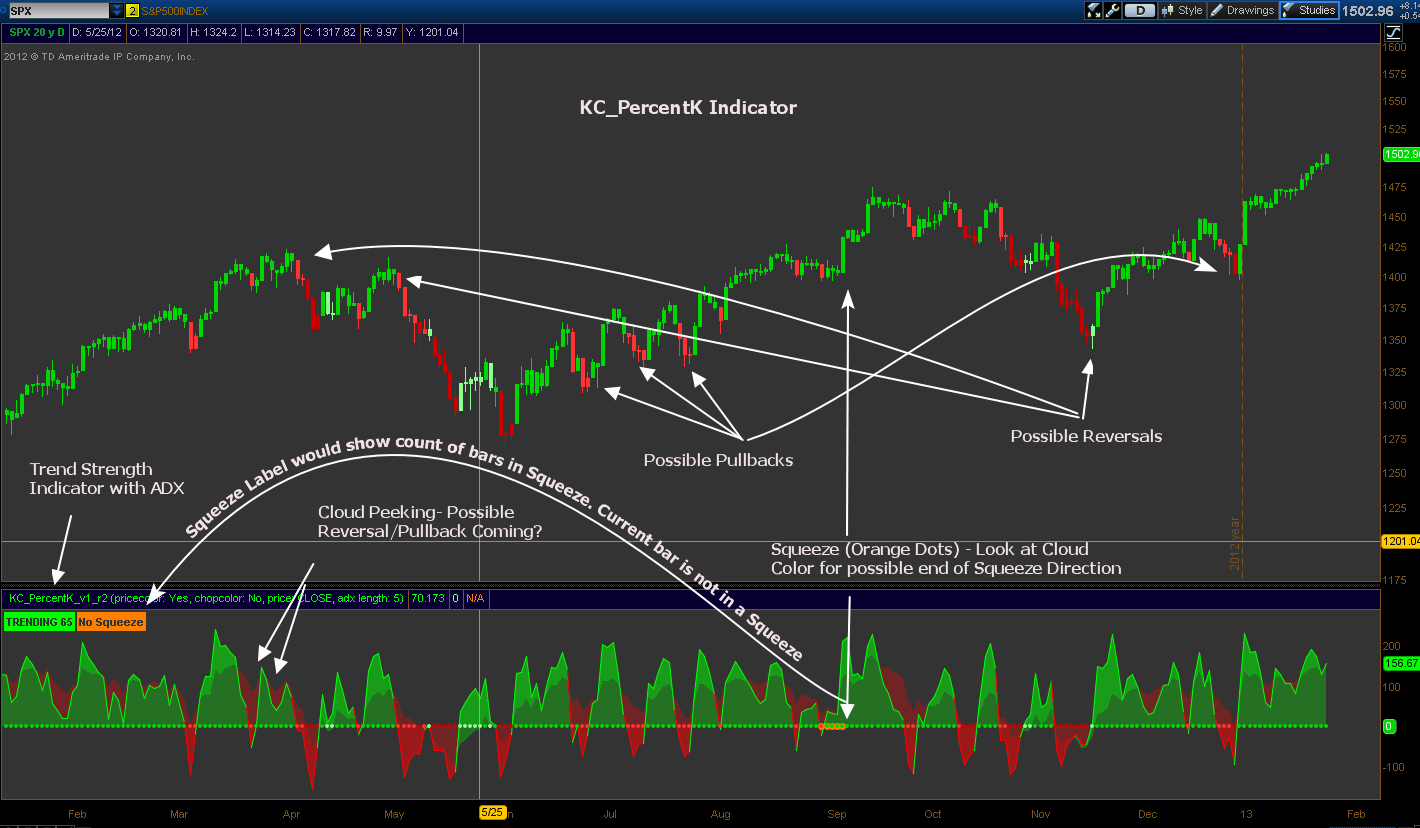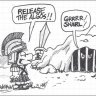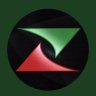# Keltner Channel Trend Indicator for ThinkorSwim

A

#### asianboy

##### New member
Found something very coolIt is a lower indicator with Price Bar Coloring options are as follows:

 - Bright_Green : Both Short term and Long term trends are Up, - Bright_Green : Both Short term and Long term trends are Up, - Bright_Red : Both Short term and Long term trends are Down, - Light_Red : Short term trend is Down and Long term trend is Up, - Yellow : If ChopColor is selected yes, then all Price Bars occurring during Choppiness periods per the Trend Strength Label (discussed below) will be colored.

### thinkScript Code

Code:
``````# KC_PercentK V1_R2

# KeltnerChannels set to similar BB PercentB

# Developed by Lar with assistance from TrendXplorer

# Trending and Squeeze Indicators provided by Mobius @My Trade

# Orange dots indicate squeeze.

# Orange Label indicates Squeeze status and number of consecutive bars being squeezed.

# The longer the squeeze is lasting may indicate a larger move when it ends.

#

# Green dots on zero line indicate trend direction is up.

# Red dots on zero line indicate trend is down.

#

# Cloud colors indicate short term price action green/red and long term

# dark_green/dark_red.

#

# Trend Strength label is worded Trending and color coded to be light_green (weak)

# and green (strong) for uptrends and light_red (weak) and red (strong) for downtrends.

# It is worded Choppy and color coded light_green/light_red for Choppiness.

#

# Coloring of Price bars can be selected:

# - Bright Green = Both Short term and Long term trends are Up,

# - Light Green = Short term trend is Up and Long term trends is Down,

# - Bright Red = Both Short term and Long term trends are Down,

# - Light Red = Short term trend is Down and Long term trend is Up.

# During uptrends, light red colors may indicate a pullback if light red cloud is

# shrinking or above -100 and then Green dots continue. Otherwise, a possible trend

# reversal is indicated. The opposite applies for downtrends.

#

# Coloring of Price bars for Choppiness can be selected. Yellow = Bars in Chop.

declare lower;

input pricecolor    = yes;

input chopcolor = no; #Hint chopcolor: Choose yes to have Price bars colored Yellow while the trend is CHOPPY.

def price = close;

def displace = 0;

def h = high;

def l = low;

def c               = close;

# Keltner Channel set similar to BollingerBands PercentB by Lar  (Longer Term Trend)

def factorKCLT = 1.500;

def lengthKCLT   = 20.000;

def shiftKCLT   = factorKCLT * AvgTrueRange(h, c, l, lengthKCLT);

def averageKCLT = SimpleMovingAvg(price, lengthKCLT);

def AvgKCLT = averageKCLT[-displace];

def Upper_BandKCLT = averageKCLT[-displace] + shiftKCLT[-displace];

def Lower_BandKCLT = averageKCLT[-displace] - shiftKCLT[-displace];

plot PercentkLT = (price - Lower_BandKCLT) / (Upper_BandKCLT - Lower_BandKCLT) * 100;

PercentkLT.AssignValueColor(if PercentkLT > 0 then Color.GREEN else Color.RED);

PercentkLT.SetLineWeight(1);

PercentkLT.Hide();

# Keltner Channel set similar to BollingerBands PercentB by Lar (Shorter Term Trend)

def factorKCST = .500;

def lengthKCST   = 6.000;

def shiftKCST   = factorKCST * AvgTrueRange(h, c, l, lengthKCST);

def averageKCST = SimpleMovingAvg(price, lengthKCST);

def AvgKCST = averageKCST[-displace];

def Upper_BandKCST = averageKCST[-displace] + shiftKCST[-displace];

def Lower_BandKCST = averageKCST[-displace] - shiftKCST[-displace];

plot PercentkST = (price - Lower_BandKCST) / (Upper_BandKCST - Lower_BandKCST) * 100;

PercentkST.AssignValueColor(if PercentkST > 0 then Color.GREEN else Color.RED);

PercentkST.SetLineWeight(1);

PercentkST.HideBubble();

# Trends Defined

# Long Term Trend

rec Uptrend = if Uptrend == 0 and PercentkLT >= 0 then 1 else if Uptrend == 1 and PercentkLT >= 0 then 1 else 0;

rec Downtrend = if Uptrend == 0 then 1 else 0;

# Short Term Trend

rec uptrendsmall = if uptrendsmall == 0 and PercentkST >= 0 then 1 else if uptrendsmall == 1 and PercentkST >= 0 then 1 else 0;

rec downtrendsmall = if uptrendsmall == 0 then 1 else 0;

# Line Definitions

def ZeroLine = if !IsNaN(price) then 0 else Double.NaN;

def HalfLine = if !IsNaN(price) then 50 else Double.NaN;

def UnitLine = if !IsNaN(price) then 100 else Double.NaN;

# Clouds

# Choppiness Indicator from Mobius @My Trade with color changes by Lar

# Indicates Trending or Chop along with current ADX level

def ADXLength    = 5; # TOS default 10

def SMALength = 8; # TOS default 8

def Lengthchop = 8;

def Signal = 3;

def Choppy = 61.8;

def MidLine = 50;

def Trending     = 38.2;

def SMA = Average(price, SMALength);

def CIB = ((Log(Sum(TrueRange(h, c, l), Lengthchop) /

(Highest(if h >= c then h else

c, Lengthchop) -

Lowest( if l <= c then l else c, Lengthchop)))

/ Log(10)) / (Log(Lengthchop) / Log(10))) * 100;

def CI = CIB;

def Mid = MidLine;

def Trend1 = Trending;

def TrendLine = 0;

# Plot of Trends

plot trend = if uptrendsmall == 1 or downtrendsmall == 1 then TrendLine else Double.NaN;

trend.SetPaintingStrategy(PaintingStrategy.POINTS);

trend.AssignValueColor(if Uptrend == 1 and uptrendsmall == 1 then createcolor(0,214,0) else if uptrendsmall == 1 and Uptrend == 0 then createcolor(138,255,138) else if Downtrend == 1 and downtrendsmall == 1 then createcolor(204,0,0) else if downtrendsmall == 1 and Downtrend == 0 then createcolor(255,51,51) else Color.YELLOW);

trend.SetLineWeight(1);

trend.HideBubble();

# Choppiness Indicator Label

AddLabel(yes, if CI > MidLine then "CHOPPY " + ADX else "TRENDING " + ADX, if CI < Trend1 then (if uptrendsmall == 1 then Color.GREEN else Color.RED) else if CI > Trend1 and CI < MidLine then (if uptrendsmall == 1 then Color.LIGHT_GREEN else Color.LIGHT_RED) else if CI > MidLine and uptrendsmall == 1 then Color.LIGHT_GREEN else Color.LIGHT_RED);

# Squeeze by Mobius @ My Trade with color mod by Lar

# Look at Cloud Color as a possible indication of direction once squeeze ends

def nK = 1.5;

def nBB = 2.0;

def lengthsqueeze = 20;

def BBHalfWidth = StDev(price, lengthsqueeze);

def KCHalfWidth = nK * AvgTrueRange(h, c, l, lengthsqueeze);

def isSqueezed  =  nBB * BBHalfWidth / KCHalfWidth < 1;

# Squeeze for Longer Term Trend Indicator

plot BBS_Ind = if isSqueezed then TrendLine else Double.NaN;

BBS_Ind.SetDefaultColor(Color.DARK_ORANGE);

BBS_Ind.SetPaintingStrategy(PaintingStrategy.POINTS);

BBS_Ind.SetLineWeight(4);

BBS_Ind.HideBubble();

# Count of Periods in consecutive squeeze

rec count = if isSqueezed then count + 1 else 0;

AddLabel(yes, if isSqueezed then Concat("Squeeze ", count) else "No Squeeze", Color.DARK_ORANGE);

# Paint Price Bars

assignPriceColor(if pricecolor==yes then if uptrendsmall==1 and CI > MidLine and chopcolor==yes then Color.yellow else if uptrendsmall==1 and uptrend==1 then createcolor(0,214,0) else if Uptrend==0 and Uptrendsmall==1 then createcolor(138,255,138) else if  Downtrendsmall==1 and CI > MidLine and chopcolor==yes then Color.yellow else if Downtrend==1 and downtrendsmall==1 then createcolor(204,0,0) else if downtrend==0 and downtrendsmall==1 then createcolor(255,51,51) else color.current else color.current);``````

Last edited by a moderator:
•A

#### ArrOne

##### New member
2019 Donor
Backtesting this now and it already seems extremely helpful, very interesting find! Thank you for sharing.

A

#### asianboy

##### New member
Backtesting this now and it already seems extremely helpful, very interesting find! Thank you for sharing.
there is scanner too on link lower comments if u want to test#### markos

##### Well-known member
VIP
Found something very coolIt is a lower indicator with Price Bar Coloring options are as follows:

 - Bright_Green : Both Short term and Long term trends are Up, - Bright_Green : Both Short term and Long term trends are Up, - Bright_Red : Both Short term and Long term trends are Down, - Light_Red : Short term trend is Down and Long term trend is Up, - Yellow : If ChopColor is selected yes, then all Price Bars occurring during Choppiness periods per the Trend Strength Label (discussed below) will be colored.

### thinkScript Code

Code:
``````# KC_PercentK V1_R2

# KeltnerChannels set to similar BB PercentB

# Developed by Lar with assistance from TrendXplorer

# Trending and Squeeze Indicators provided by Mobius @My Trade

# Orange dots indicate squeeze.

# Orange Label indicates Squeeze status and number of consecutive bars being squeezed.

# The longer the squeeze is lasting may indicate a larger move when it ends.

#

# Green dots on zero line indicate trend direction is up.

# Red dots on zero line indicate trend is down.

#

# Cloud colors indicate short term price action green/red and long term

# dark_green/dark_red.

#

# Trend Strength label is worded Trending and color coded to be light_green (weak)

# and green (strong) for uptrends and light_red (weak) and red (strong) for downtrends.

# It is worded Choppy and color coded light_green/light_red for Choppiness.

#

# Coloring of Price bars can be selected:

# - Bright Green = Both Short term and Long term trends are Up,

# - Light Green = Short term trend is Up and Long term trends is Down,

# - Bright Red = Both Short term and Long term trends are Down,

# - Light Red = Short term trend is Down and Long term trend is Up.

# During uptrends, light red colors may indicate a pullback if light red cloud is

# shrinking or above -100 and then Green dots continue. Otherwise, a possible trend

# reversal is indicated. The opposite applies for downtrends.

#

# Coloring of Price bars for Choppiness can be selected. Yellow = Bars in Chop.

declare lower;

input pricecolor    = yes;

input chopcolor = no; #Hint chopcolor: Choose yes to have Price bars colored Yellow while the trend is CHOPPY.

def price = close;

def displace = 0;

def h = high;

def l = low;

def c               = close;

# Keltner Channel set similar to BollingerBands PercentB by Lar  (Longer Term Trend)

def factorKCLT = 1.500;

def lengthKCLT   = 20.000;

def shiftKCLT   = factorKCLT * AvgTrueRange(h, c, l, lengthKCLT);

def averageKCLT = SimpleMovingAvg(price, lengthKCLT);

def AvgKCLT = averageKCLT[-displace];

def Upper_BandKCLT = averageKCLT[-displace] + shiftKCLT[-displace];

def Lower_BandKCLT = averageKCLT[-displace] - shiftKCLT[-displace];

plot PercentkLT = (price - Lower_BandKCLT) / (Upper_BandKCLT - Lower_BandKCLT) * 100;

PercentkLT.AssignValueColor(if PercentkLT > 0 then Color.GREEN else Color.RED);

PercentkLT.SetLineWeight(1);

PercentkLT.Hide();

# Keltner Channel set similar to BollingerBands PercentB by Lar (Shorter Term Trend)

def factorKCST = .500;

def lengthKCST   = 6.000;

def shiftKCST   = factorKCST * AvgTrueRange(h, c, l, lengthKCST);

def averageKCST = SimpleMovingAvg(price, lengthKCST);

def AvgKCST = averageKCST[-displace];

def Upper_BandKCST = averageKCST[-displace] + shiftKCST[-displace];

def Lower_BandKCST = averageKCST[-displace] - shiftKCST[-displace];

plot PercentkST = (price - Lower_BandKCST) / (Upper_BandKCST - Lower_BandKCST) * 100;

PercentkST.AssignValueColor(if PercentkST > 0 then Color.GREEN else Color.RED);

PercentkST.SetLineWeight(1);

PercentkST.HideBubble();

# Trends Defined

# Long Term Trend

rec Uptrend = if Uptrend == 0 and PercentkLT >= 0 then 1 else if Uptrend == 1 and PercentkLT >= 0 then 1 else 0;

rec Downtrend = if Uptrend == 0 then 1 else 0;

# Short Term Trend

rec uptrendsmall = if uptrendsmall == 0 and PercentkST >= 0 then 1 else if uptrendsmall == 1 and PercentkST >= 0 then 1 else 0;

rec downtrendsmall = if uptrendsmall == 0 then 1 else 0;

# Line Definitions

def ZeroLine = if !IsNaN(price) then 0 else Double.NaN;

def HalfLine = if !IsNaN(price) then 50 else Double.NaN;

def UnitLine = if !IsNaN(price) then 100 else Double.NaN;

# Clouds

# Choppiness Indicator from Mobius @My Trade with color changes by Lar

# Indicates Trending or Chop along with current ADX level

def ADXLength    = 5; # TOS default 10

def SMALength = 8; # TOS default 8

def Lengthchop = 8;

def Signal = 3;

def Choppy = 61.8;

def MidLine = 50;

def Trending     = 38.2;

def SMA = Average(price, SMALength);

def CIB = ((Log(Sum(TrueRange(h, c, l), Lengthchop) /

(Highest(if h >= c then h else

c, Lengthchop) -

Lowest( if l <= c then l else c, Lengthchop)))

/ Log(10)) / (Log(Lengthchop) / Log(10))) * 100;

def CI = CIB;

def Mid = MidLine;

def Trend1 = Trending;

def TrendLine = 0;

# Plot of Trends

plot trend = if uptrendsmall == 1 or downtrendsmall == 1 then TrendLine else Double.NaN;

trend.SetPaintingStrategy(PaintingStrategy.POINTS);

trend.AssignValueColor(if Uptrend == 1 and uptrendsmall == 1 then createcolor(0,214,0) else if uptrendsmall == 1 and Uptrend == 0 then createcolor(138,255,138) else if Downtrend == 1 and downtrendsmall == 1 then createcolor(204,0,0) else if downtrendsmall == 1 and Downtrend == 0 then createcolor(255,51,51) else Color.YELLOW);

trend.SetLineWeight(1);

trend.HideBubble();

# Choppiness Indicator Label

AddLabel(yes, if CI > MidLine then "CHOPPY " + ADX else "TRENDING " + ADX, if CI < Trend1 then (if uptrendsmall == 1 then Color.GREEN else Color.RED) else if CI > Trend1 and CI < MidLine then (if uptrendsmall == 1 then Color.LIGHT_GREEN else Color.LIGHT_RED) else if CI > MidLine and uptrendsmall == 1 then Color.LIGHT_GREEN else Color.LIGHT_RED);

# Squeeze by Mobius @ My Trade with color mod by Lar

# Look at Cloud Color as a possible indication of direction once squeeze ends

def nK = 1.5;

def nBB = 2.0;

def lengthsqueeze = 20;

def BBHalfWidth = StDev(price, lengthsqueeze);

def KCHalfWidth = nK * AvgTrueRange(h, c, l, lengthsqueeze);

def isSqueezed  =  nBB * BBHalfWidth / KCHalfWidth < 1;

# Squeeze for Longer Term Trend Indicator

plot BBS_Ind = if isSqueezed then TrendLine else Double.NaN;

BBS_Ind.SetDefaultColor(Color.DARK_ORANGE);

BBS_Ind.SetPaintingStrategy(PaintingStrategy.POINTS);

BBS_Ind.SetLineWeight(4);

BBS_Ind.HideBubble();

# Count of Periods in consecutive squeeze

rec count = if isSqueezed then count + 1 else 0;

AddLabel(yes, if isSqueezed then Concat("Squeeze ", count) else "No Squeeze", Color.DARK_ORANGE);

# Paint Price Bars

assignPriceColor(if pricecolor==yes then if uptrendsmall==1 and CI > MidLine and chopcolor==yes then Color.yellow else if uptrendsmall==1 and uptrend==1 then createcolor(0,214,0) else if Uptrend==0 and Uptrendsmall==1 then createcolor(138,255,138) else if  Downtrendsmall==1 and CI > MidLine and chopcolor==yes then Color.yellow else if Downtrend==1 and downtrendsmall==1 then createcolor(204,0,0) else if downtrend==0 and downtrendsmall==1 then createcolor(255,51,51) else color.current else color.current);``````

Thank you for sharing. I think LAR mis-used the Chop Indicator from Mobius, but if it works for you, that's great.#### Billions

##### Active member
2019 Donor
VIP
@asianboy what timeframe can this be used on?

A

#### asianboy

##### New member
Billions it work any time timeframe. i use 5min and 15 min for squeeze and pullback.#### Billions

##### Active member
2019 Donor
VIP
@asianboy thanks I'm trying to figure It out. Anything others tweets I would appreciate or what works best what signs you wait for work best. Thanks!

T

##### Active member
2019 Donor
Ben is possible to make this into a MTF multi time frame indicator ?

T

##### Active member
2019 Donor
Can the code be adjusted for MTF multi time ?

Last edited:
J

#### JBL73

##### New member
What do the numbers next to Choppy and Squeeze indicate? I looked at the pic again and it says on it. : )

Last edited:
A

#### asianboy

##### New member
If someone can add Bollinger Band Wave it will be nice#### horserider

##### Well-known member
VIP
@asianboy Please, what is a Bollinger Band Wave ?
From what I can see it already is using BB and Keltner Channels to determine the squeeze.

T

##### Active member
2019 Donor
If the MTF can be added we could have a good entry strategy for the reversal setup, is there anyone that can help adding the aggregation?#### horserider

VIP
T

##### Active member
2019 Donor#### Billions

##### Active member
2019 Donor
VIP

•T

##### Active member
2019 Donor
It only has one line verse the two clouds, any way to get it to function the same?#### Billions

##### Active member
2019 Donor
VIP
It only has one line verse the two clouds, any way to get it to function the same?
I used it on 5 min yesterday it worked great

T

##### Active member
2019 Donor
I used it on 5 min yesterday it worked great
the regular one works i was referring to the new one with the MTF aggregation that was added, the new one only has one line verse the two clouds, i wanted to see if the MTF could function like the original one#### Billions

##### Active member
2019 Donor
VIP
the regular one works i was referring to the new one with the MTF aggregation that was added, the new one only has one line verse the two clouds, i wanted to see if the MTF could function like the original one
Oh yes I see what you mean